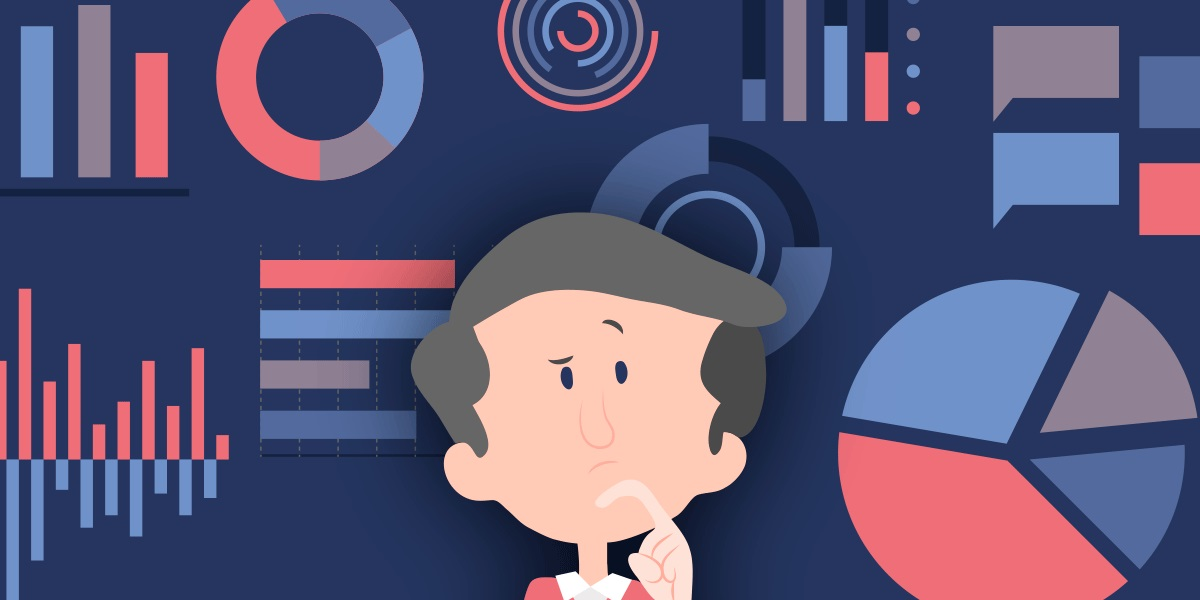# Understanding Data And Its TypesWhen we are surrounded by such a huge amount of data and even the slightest improvement in data science requires data, these data must be divided into some categories. So, speaking at the uppermost level, the data is divided into two kinds, Quantitative and Qualitative Data. Let’s discuss these two types of data in detail.

• Quantitative Data: The kind of data that can be measured equitably and handle numbers such as length, width, height, prices, humidity, temperature, area, and volume.
• Qualitative Data: As the name signifies, it deals with quality i.e., the attributes and descriptors that are not easily measured. It is observed in a subjective aspect like color, texture, attractiveness, etc.

In general, if the data is measured and assigned some number or value to it, it is a quantitative data whereas if the data generated is classified according to its characteristics, it is a qualitative data. But so far this is about the highest level of data, there exists a variety of quantitative and qualitative data.

Types of Quantitative Data

Basically, quantitative data are of two types and they are also called numeric data, those are Discrete and Continuous.

• Discrete data are those data that cannot be made more precise. The data which have a fixed integer count like the number of people in a family since the count can only be an integer number (obviously you cannot have 4.5 members in a family). So that’s why these kinds of data and figures are referred to as discrete.
• Continuous Data are those which are easily precise i.e. can be reduced and divided at more finer levels. These data can have decimals in between figures like the height of a person at more precise scale levels (millimeters, centimeters, meters). That’s why height is classified as an example of continuous data. Continuous data are preferred where the accuracy and hypothesis tests are carried out.

Types of Qualitative Data

The qualitative approach of data can be divided into three types: Binomial, Ordinal, and Nominal data.

• Binomial data or binary data classifies data into either of the two states, one or zero, true or false, good or bad, accept or reject. The use of this type of data is that a statistical model can be developed so that a prediction can be made on how often a ‘true’ value is expected or vice-versa.
• Ordinal data or ordered data are the data in which categories are being assigned certain items that contains particular implicit order. A good example of this can be a survey form wherein you are asked to rate an item on a scale of 1 to 10 (10 being the best and 1 being the worst).
• Nominal data or unordered data are those in which categories are being assigned individual items that do not contain any implicit value or order. This kind of data is useful for statistical purposes to see the differences in the attributes of each item.

Resource Box

To grow in the field of Data Science, one must know the basics of data, its storage methods and the types. For this, a data science course in hyderabad is available at 360digitmg to get anyone through all data related facts.